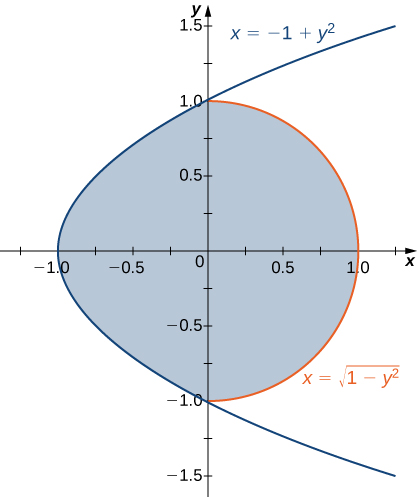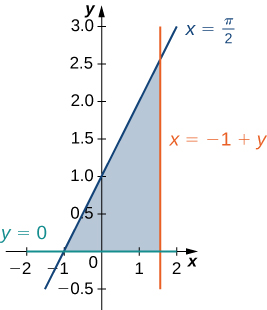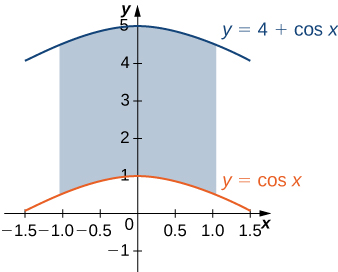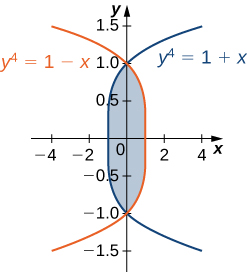$$\newcommand{\id}{\mathrm{id}}$$ $$\newcommand{\Span}{\mathrm{span}}$$ $$\newcommand{\kernel}{\mathrm{null}\,}$$ $$\newcommand{\range}{\mathrm{range}\,}$$ $$\newcommand{\RealPart}{\mathrm{Re}}$$ $$\newcommand{\ImaginaryPart}{\mathrm{Im}}$$ $$\newcommand{\Argument}{\mathrm{Arg}}$$ $$\newcommand{\norm}{\| #1 \|}$$ $$\newcommand{\inner}{\langle #1, #2 \rangle}$$ $$\newcommand{\Span}{\mathrm{span}}$$

# 14.2bE: Double Integrals Part 2 (Exercises)

$$\newcommand{\vecs}{\overset { \rightharpoonup} {\mathbf{#1}} }$$ $$\newcommand{\vecd}{\overset{-\!-\!\rightharpoonup}{\vphantom{a}\smash {#1}}}$$$$\newcommand{\id}{\mathrm{id}}$$ $$\newcommand{\Span}{\mathrm{span}}$$ $$\newcommand{\kernel}{\mathrm{null}\,}$$ $$\newcommand{\range}{\mathrm{range}\,}$$ $$\newcommand{\RealPart}{\mathrm{Re}}$$ $$\newcommand{\ImaginaryPart}{\mathrm{Im}}$$ $$\newcommand{\Argument}{\mathrm{Arg}}$$ $$\newcommand{\norm}{\| #1 \|}$$ $$\newcommand{\inner}{\langle #1, #2 \rangle}$$ $$\newcommand{\Span}{\mathrm{span}}$$ $$\newcommand{\id}{\mathrm{id}}$$ $$\newcommand{\Span}{\mathrm{span}}$$ $$\newcommand{\kernel}{\mathrm{null}\,}$$ $$\newcommand{\range}{\mathrm{range}\,}$$ $$\newcommand{\RealPart}{\mathrm{Re}}$$ $$\newcommand{\ImaginaryPart}{\mathrm{Im}}$$ $$\newcommand{\Argument}{\mathrm{Arg}}$$ $$\newcommand{\norm}{\| #1 \|}$$ $$\newcommand{\inner}{\langle #1, #2 \rangle}$$ $$\newcommand{\Span}{\mathrm{span}}$$

1) The region $$D$$ bounded by $$y = x^3, \space y = x^3 + 1, \space x = 0,$$ and $$x = 1$$ as given in the following figure.a. Classify this region as vertically simple (Type I) or horizontally simple (Type II).

Type:
Type I but not Type II

b. Find the area of the region $$D$$.

c. Find the average value of the function $$f(x,y) = 3xy$$ on the region graphed in the previous exercise.

$$\frac{27}{20}$$

2) The region $$D$$ bounded by $$y = \sin x, \space y = 1 + \sin x, \space x = 0$$, and $$x = \frac{\pi}{2}$$ as given in the following figure.a. Classify this region as vertically simple (Type I) or horizontally simple (Type II).

Type:
Type I but not Type II

b. Find the area of the region $$D$$.

$$\frac{\pi}{2}\, \text{units}^2$$

c. Find the average value of the function $$f(x,y) = \cos x$$ on the region $$D$$.

3) The region $$D$$ bounded by $$x = y^2 - 1$$ and $$x = \sqrt{1 - y^2}$$ as given in the following figure.a. Classify this region as vertically simple (Type I) or horizontally simple (Type II).

Type:
Type II but not Type I

b. Find the volume of the solid under the graph of the function $$f(x,y) = xy + 1$$ and above the region $$D$$.

$$\frac{1}{6}(8 + 3\pi)\, \text{units}^3$$

4) The region $$D$$ bounded by $$y = 0, \space x = -10 + y,$$ and $$x = 10 - y$$ as given in the following figure.a. Classify this region as vertically simple (Type I) or horizontally simple (Type II).

Type:
Type II but not Type I

b. Find the volume of the solid under the graph of the function $$f(x,y) = x + y$$ and above the region in the figure from the previous exercise.

$$\frac{1000}{3}\, \text{units}^3$$

5) The region $$D$$ bounded by $$y = 0, \space x = y - 1, \space x = \frac{\pi}{2}$$ as given in the following figure.Classify this region as vertically simple (Type I) or horizontally simple (Type II).

Type:
Type I and Type II

6) The region $$D$$ bounded by $$y = 0$$ and $$y = x^2 - 1$$ as given in the following figure.Classify this region as vertically simple (Type I) or horizontally simple (Type II).

Type:
Type I and Type II

7) Let $$D$$ be the region bounded by the curves of equations $$y = \cos x$$ and $$y = 4 - x^2$$ and the $$x$$-axis. Explain why $$D$$ is neither of Type I nor II.

The region $$D$$ is not of Type I: it does not lie between two vertical lines and the graphs of two continuous functions $$g_1(x)$$ and $$g_2(x)$$. The region is not of Type II: it does not lie between two horizontal lines and the graphs of two continuous functions $$h_1(y)$$ and $$h_2(y)$$.

8) Let $$D$$ be the region bounded by the curves of equations $$y = x, \space y = -x$$ and $$y = 2 - x^2$$. Explain why $$D$$ is neither of Type I nor II.

In exercises 9 - 14, evaluate the double integral $$\displaystyle \iint_D f(x,y) \,dA$$ over the region $$D$$.

9) $$f(x,y) = 1$$ and

$$D = \big\{(x,y)| \, 0 \leq x \leq \frac{\pi}{2}, \space \sin x \leq y \leq 1 + \sin x \big\}$$

$$\frac{\pi}{2}$$

10) $$f(x,y) = 2$$ and

$$D = \big\{(x,y)| \, 0 \leq y \leq 1, \space y - 1 \leq x \leq \arccos y \big\}$$

11) $$f(x,y) = xy$$ and

$$D = \big\{(x,y)| \, -1 \leq y \leq 1, \space y^2 - 1 \leq x \leq \sqrt{1 - y^2} \big\}$$

$$0$$

12) $$f(x,y) = \sin y$$ and $$D$$ is the triangular region with vertices $$(0,0), \space (0,3)$$, and $$(3,0)$$

13) $$f(x,y) = -x + 1$$ and $$D$$ is the triangular region with vertices $$(0,0), \space (0,2)$$, and $$(2,2)$$

$$\frac{2}{3}$$

14) $$f(x,y) = 2x + 4y$$ and

$$D = \big\{(x,y)|\, 0 \leq x \leq 1, \space x^3 \leq y \leq x^3 + 1 \big\}$$

In exercises 15 - 20, evaluate the iterated integrals.

15) $$\displaystyle \int_0^1 \int_{2\sqrt{x}}^{2\sqrt{x}+1} (xy + 1) \,dy \space dx$$

$$\frac{41}{20}$$

16) $$\displaystyle \int_0^3 \int_{2x}^{3x} (x + y^2) \,dy \space dx$$

17) $$\displaystyle \int_1^2 \int_{-u^2-1}^{-u} (8 uv) \,dv \space du$$

$$-63$$

18) $$\displaystyle \int_e^{e^2} \int_{\ln u}^2 (v + \ln u) \,dv \space du$$

19) $$\displaystyle \int_0^{1/2} \int_{-\sqrt{1-4y^2}}^{\sqrt{1-4y^2}} 4 \,dx \space dy$$

$$\pi$$

20) $$\displaystyle \int_0^1 \int_{-\sqrt{1-y^2}}^{\sqrt{1-y^2}} (2x + 4y^3) \,dx \space dy$$

21) Let $$D$$ be the region bounded by $$y = 1 - x^2, \space y = 4 - x^2$$, and the $$x$$- and $$y$$-axes.

a. Show that $$\displaystyle \iint_D x\,dA = \int_0^1 \int_{1-x^2}^{4-x^2} x \space dy \space dx + \int_1^2 \int_0^{4-x^2} x \space dy \space dx$$ by dividing the region $$D$$ into two regions of Type I.

b. Evaluate the integral $$\displaystyle \iint_D x \,dA.$$

22) Let $$D$$ be the region bounded by $$y = 1, \space y = x, \space y = \ln x$$, and the $$x$$-axis.

a. Show that $$\displaystyle \iint_D y^2 \,dA = \int_{-1}^0 \int_{-x}^{2-x^2} y^2 dy \space dx + \int_0^1 \int_x^{2-x^2} y^2 dy \space dx$$ by dividing the region $$D$$ into two regions of Type I, where $$D = \big\{(x,y)\,|\,y \geq x, y \geq -x, \space y \leq 2-x^2\big\}$$.

b. Evaluate the integral $$\displaystyle \iint_D y^2 \,dA.$$

23) Let $$D$$ be the region bounded by $$y = x^2$$, $$y = x + 2$$, and $$y = -x$$.

a. Show that $$\displaystyle \iint_D x \, dA = \int_0^1 \int_{-y}^{\sqrt{y}} x \space dx \space dy + \int_1^4 \int_{y-2}^{\sqrt{y}} x \space dx \space dy$$ by dividing the region $$D$$ into two regions of Type II, where $$D = \big\{(x,y)\,|\,y \geq x^2, \space y \geq -x, \space y \leq x + 2\big\}$$.

b. Evaluate the integral $$\displaystyle \iint_D x \,dA.$$

b. $$\frac{8}{12}$$

24) The region $$D$$ bounded by $$x = 0, y = x^5 + 1$$, and $$y = 3 - x^2$$ is shown in the following figure. Find the area $$A(D)$$ of the region $$D$$.25) The region $$D$$ bounded by $$y = \cos x, \space y = 4 + \cos x$$, and $$x = \pm \frac{\pi}{3}$$ is shown in the following figure. Find the area $$A(D)$$ of the region $$D$$.$$\frac{8\pi}{3}$$

26) Find the area $$A(D)$$ of the region $$D = \big\{(x,y)| \, y \geq 1 - x^2, y \leq 4 - x^2, \space y \geq 0, \space x \geq 0 \big\}$$.

27) Let $$D$$ be the region bounded by $$y = 1, \space y = x, \space y = \ln x$$, and the $$x$$-axis. Find the area $$A(D)$$ of the region $$D$$.

$$\left(e - \frac{3}{2}\right)\, \text{units}^2$$

28) Find the average value of the function $$f(x,y) = \sin y$$ on the triangular region with vertices $$(0,0), \space (0,3)$$, and $$(3,0)$$.

29) Find the average value of the function $$f(x,y) = -x + 1$$ on the triangular region with vertices $$(0,0), \space (0,2)$$, and $$(2,2)$$.

The average value of $$f$$ on this triangular region is $$\frac{1}{3}.$$

In exercises 30 - 33, change the order of integration and evaluate the integral.

30) $$\displaystyle \int_{-1}^{\pi/2} \int_0^{x+1} \sin x \, dy \, dx$$

31) $$\displaystyle \int_0^1 \int_{x-1}^{1-x} x \, dy \, dx$$

$$\displaystyle \int_0^1 \int_{x-1}^{1-x} x \space dy \space dx = \int_{-1}^0 \int_0^{y+1} x \space dx \space dy + \int_0^1 \int_0^{1-y} x \space dx \space dy = \frac{1}{3}$$

32) $$\displaystyle \int_{-1}^0 \int_{-\sqrt{y+1}}^{\sqrt{y+1}} y^2 dx \space dy$$

33) $$\displaystyle \int_{-1/2}^{1/2} \int_{-\sqrt{y^2+1}}^{\sqrt{y^2+1}} y \space dx \space dy$$

$$\displaystyle \int_{-1/2}^{1/2} \int_{-\sqrt{y^2+1}}^{\sqrt{y^2+1}} y \space dx \space dy = \int_1^2 \int_{-\sqrt{x^2-1}}^{\sqrt{x^2-1}} y \space dy \space dx = 0$$

34) The region $$D$$ is shown in the following figure. Evaluate the double integral $$\displaystyle \iint_D (x^2 + y) \,dA$$ by using the easier order of integration.35) The region $$D$$ is shown in the following figure. Evaluate the double integral $$\displaystyle \iint_D (x^2 - y^2) \,dA$$ by using the easier order of integration.$$\displaystyle \iint_D (x^2 - y^2) dA = \int_{-1}^1 \int_{y^4-1}^{1-y^4} (x^2 - y^2)dx \space dy = \frac{464}{4095}$$

36) Find the volume of the solid under the surface $$z = 2x + y^2$$ and above the region bounded by $$y = x^5$$ and $$y = x$$.

37) Find the volume of the solid under the plane $$z = 3x + y$$ and above the region determined by $$y = x^7$$ and $$y = x$$.

$$\frac{4}{5}\, \text{units}^3$$

38) Find the volume of the solid under the plane $$z = 3x + y$$ and above the region bounded by $$x = \tan y, \space x = -\tan y$$, and $$x = 1$$.

39) Find the volume of the solid under the surface $$z = x^3$$ and above the plane region bounded by $$x = \sin y, \space x = -\sin y$$, and $$x = 1$$.

$$\frac{5\pi}{32}\, \text{units}^3$$

40) Let $$g$$ be a positive, increasing, and differentiable function on the interval $$[a,b]$$. Show that the volume of the solid under the surface $$z = g'(x)$$ and above the region bounded by $$y = 0, \space y = g(x), \space x = a$$, and $$x = b$$ is given by $$\frac{1}{2}(g^2 (b) - g^2 (a))$$.

41) Let $$g$$ be a positive, increasing, and differentiable function on the interval $$[a,b]$$ and let $$k$$ be a positive real number. Show that the volume of the solid under the surface $$z = g'(x)$$ and above the region bounded by $$y = g(x), \space y = g(x) + k, \space x = a$$, and $$x = b$$ is given by $$k(g(b) - g(a)).$$

42) Find the volume of the solid situated in the first octant and determined by the planes $$z = 2$$, $$z = 0, \space x + y = 1, \space x = 0$$, and $$y = 0$$.

43) Find the volume of the solid situated in the first octant and bounded by the planes $$x + 2y = 1$$, $$x = 0, \space z = 4$$, and $$z = 0$$.

$$1\, \text{units}^3$$

44) Find the volume of the solid bounded by the planes $$x + y = 1, \space x - y = 1, \space x = 0, \space z = 0$$, and $$z = 10$$.

45) Find the volume of the solid bounded by the planes $$x + y = 1, \space x - y = 1, \space x + y = -1\space x - y = -1, \space z = 1$$, and $$z = 0$$

$$2\, \text{units}^3$$

46) Let $$S_1$$ and $$S_2$$ be the solids situated in the first octant under the planes $$x + y + z = 1$$ and $$x + y + 2z = 1$$ respectively, and let $$S$$ be the solid situated between $$S_1, \space S_2, \space x = 0$$, and $$y = 0$$.

1. Find the volume of the solid $$S_1$$.
2. Find the volume of the solid $$S_2$$.
3. Find the volume of the solid $$S$$ by subtracting the volumes of the solids $$S_1$$ and $$S_2$$.

47) Let $$S_1$$ and $$S_2$$ be the solids situated in the first octant under the planes $$2x + 2y + z = 2$$ and $$x + y + z = 1$$ respectively, and let $$S$$ be the solid situated between $$S_1, \space S_2, \space x = 0$$, and $$y = 0$$.

1. Find the volume of the solid $$S_1$$.
2. Find the volume of the solid $$S_2$$.
3. Find the volume of the solid $$S$$ by subtracting the volumes of the solids $$S_1$$ and $$S_2$$.
a. $$\frac{1}{3}\, \text{units}^3$$
b. $$\frac{1}{6}\, \text{units}^3$$
c. $$\frac{1}{6}\, \text{units}^3$$

48) Let $$S_1$$ and $$S_2$$ be the solids situated in the first octant under the plane $$x + y + z = 2$$ and under the sphere $$x^2 + y^2 + z^2 = 4$$, respectively. If the volume of the solid $$S_2$$ is $$\frac{4\pi}{3}$$ determine the volume of the solid $$S$$ situated between $$S_1$$ and $$S_2$$ by subtracting the volumes of these solids.

49) Let $$S_1$$ and $$S_2$$ be the solids situated in the first octant under the plane $$x + y + z = 2$$ and bounded by the cylinder $$x^2 + y^2 = 4$$, respectively.

1. Find the volume of the solid $$S_1$$.
2. Find the volume of the solid $$S_2$$.
3. Find the volume of the solid $$S$$ situated between $$S_1$$ and $$S_2$$ by subtracting the volumes of the solids $$S_1$$ and $$S_2$$.
a. $$\frac{4}{3}\, \text{units}^3$$
b. $$2\pi\, \text{units}^3$$
c. $$\frac{6\pi - 4}{3}\, \text{units}^3$$

50) [T] The following figure shows the region $$D$$ bounded by the curves $$y = \sin x, \space x = 0$$, and $$y = x^4$$. Use a graphing calculator or CAS to find the $$x$$-coordinates of the intersection points of the curves and to determine the area of the region $$D$$. Round your answers to six decimal places.51) [T] The region $$D$$ bounded by the curves $$y = \cos x, \space x = 0$$, and $$y = x^3$$ is shown in the following figure. Use a graphing calculator or CAS to find the $$x$$-coordinates of the intersection points of the curves and to determine the area of the region $$D$$. Round your answers to six decimal places.0 and 0.865474; $$A(D) = 0.621135\, \text{units}^3$$

52) Suppose that $$(X,Y)$$ is the outcome of an experiment that must occur in a particular region $$S$$ in the $$xy$$-plane. In this context, the region $$S$$ is called the sample space of the experiment and $$X$$ and $$Y$$ are random variables. If $$D$$ is a region included in $$S$$, then the probability of $$(X,Y)$$ being in $$D$$ is defined as $$P[(X,Y) \in D] = \iint_D p(x,y)dx \space dy$$, where $$p(x,y)$$ is the joint probability density of the experiment. Here, $$p(x,y)$$ is a nonnegative function for which $$\iint_S p(x,y) dx \space dy = 1$$. Assume that a point $$(X,Y)$$ is chosen arbitrarily in the square $$[0,3] \times [0,3]$$ with the probability density

$p(x,y) = \frac{1}{9} (x,y) \in [0,3] \times [0,3],\nonumber$

$p(x,y) = 0 \space \text{otherwise}\nonumber$

Find the probability that the point $$(X,Y)$$ is inside the unit square and interpret the result.

53) Consider $$X$$ and $$Y$$ two random variables of probability densities $$p_1(x)$$ and $$p_2(x)$$, respectively. The random variables $$X$$ and $$Y$$ are said to be independent if their joint density function is given by $$p_(x,y) = p_1(x)p_2(y)$$. At a drive-thru restaurant, customers spend, on average, 3 minutes placing their orders and an additional 5 minutes paying for and picking up their meals. Assume that placing the order and paying for/picking up the meal are two independent events $$X$$ and $$Y$$. If the waiting times are modeled by the exponential probability densities

$p_1(x) = \frac{1}{3}e^{-x/3} \space x\geq 0,\nonumber$

$p_1(x) = 0 \space \text{otherwise}\nonumber$

$p_2(y) = \frac{1}{5} e^{-y/5} \space y \geq 0\nonumber$

$p_2(y) = 0 \space \text{otherwise}\nonumber$

respectively, the probability that a customer will spend less than 6 minutes in the drive-thru line is given by $$P[X + Y \leq 6] = \iint_D p(x,y) dx \space dy$$, where $$D = {(x,y)|x \geq 0, \space y \geq 0, \space x + y \leq 6}$$. Find $$P[X + Y \leq 6]$$ and interpret the result.

$$P[X + Y \leq 6] = 1 + \frac{3}{2e^2} - \frac{5}{e^{6/5}} \approx 0.45$$; there is a $$45\%$$ chance that a customer will spend $$6$$ minutes in the drive-thru line.

54) [T] The Reuleaux triangle consists of an equilateral triangle and three regions, each of them bounded by a side of the triangle and an arc of a circle of radius s centered at the opposite vertex of the triangle. Show that the area of the Reuleaux triangle in the following figure of side length $$s$$ is $$\frac{s^2}{2}(\pi - \sqrt{3})$$.55) [T] Show that the area of the lunes of Alhazen, the two blue lunes in the following figure, is the same as the area of the right triangle $$ABC.$$ The outer boundaries of the lunes are semicircles of diameters $$AB$$ and $$AC$$ respectively, and the inner boundaries are formed by the circumcircle of the triangle $$ABC$$.